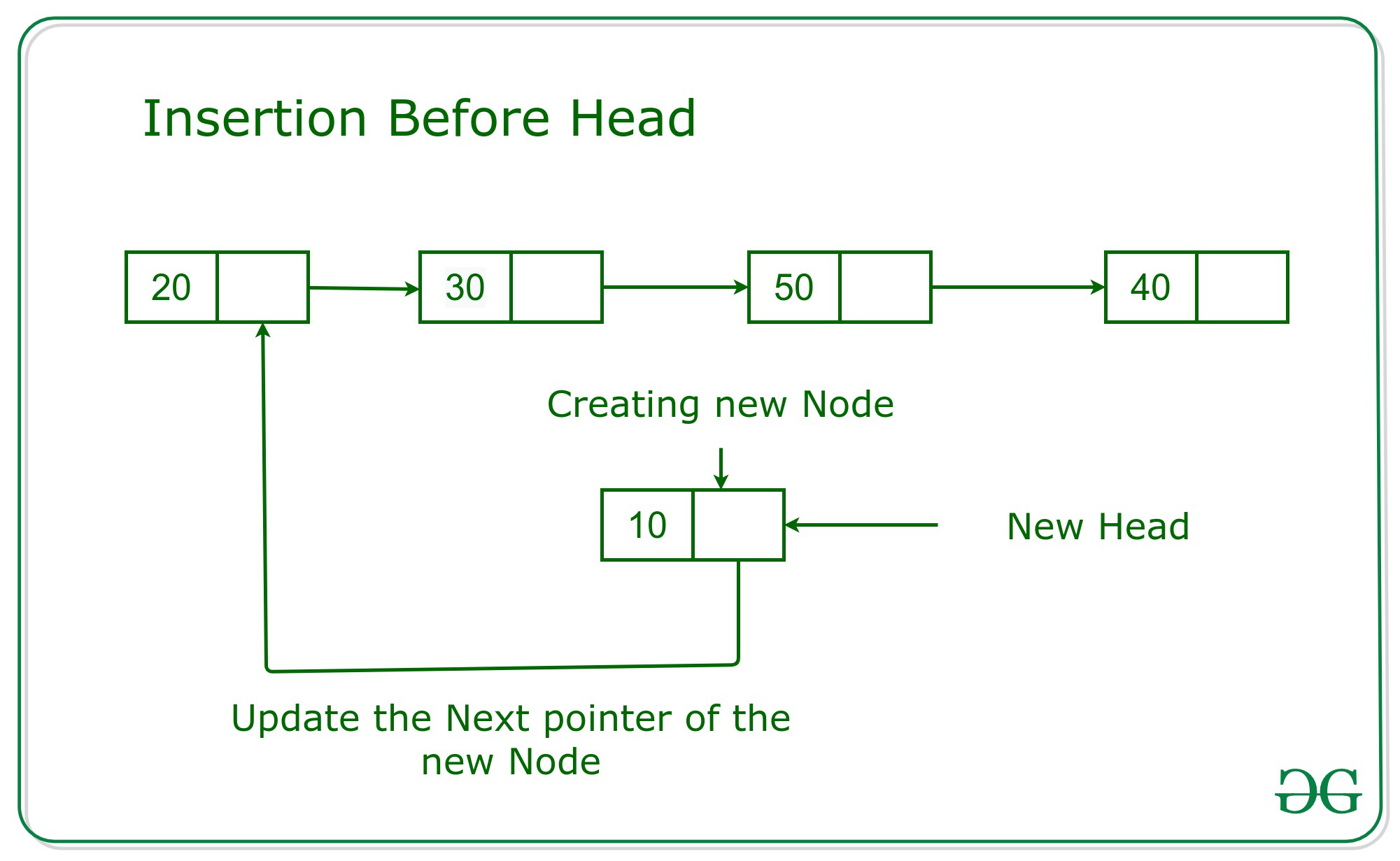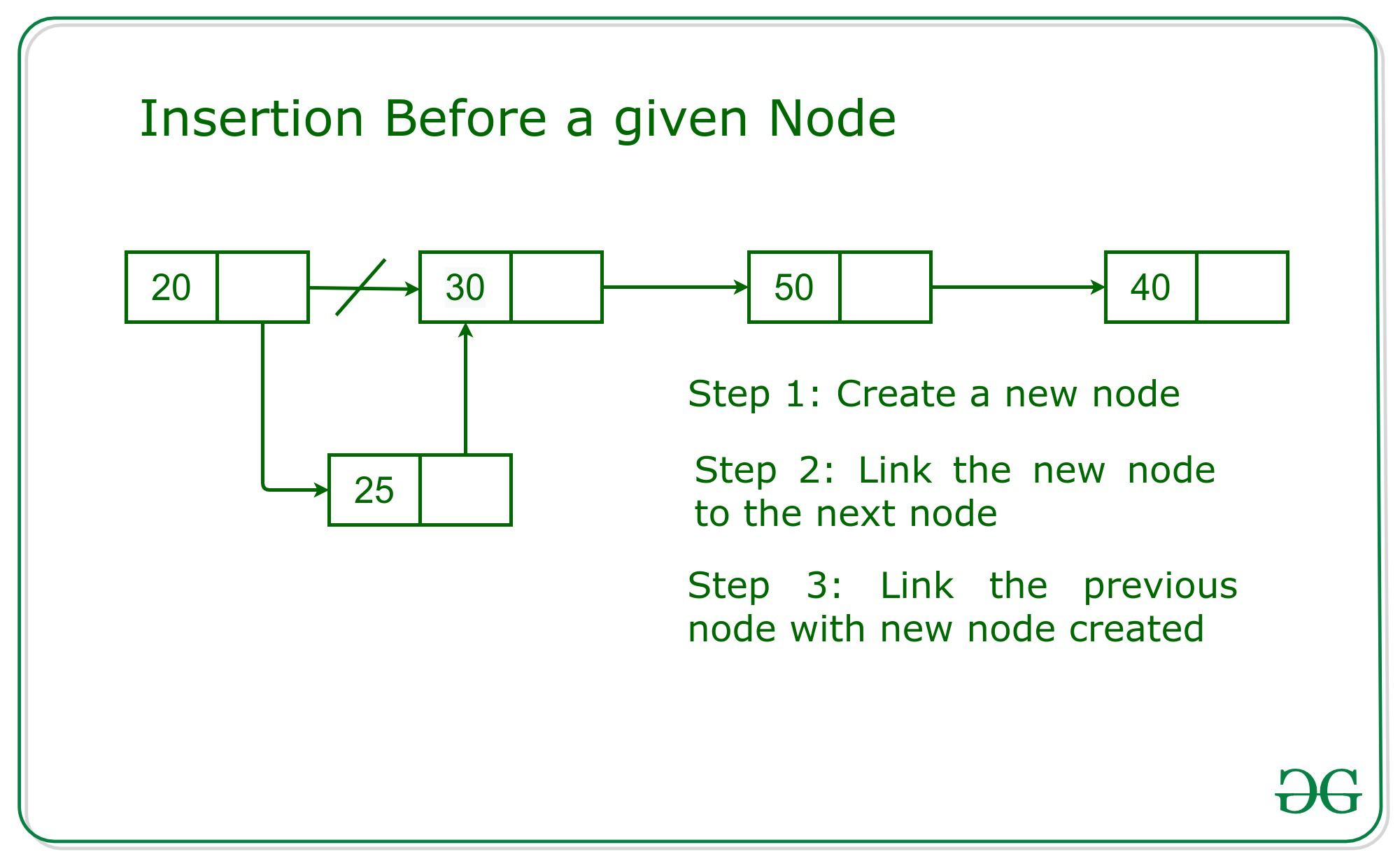# Insert a node in Linked List before a given node

Given a node of Linked List N and a value K, the task is to insert the node with value K in the linked list before the given node N.

Structure of the Node:

## C++

 `// Structure of Node` `struct` `Node {` `    ``int` `data;` `    ``Node* next;`   `    ``// Constructor of Node` `    ``Node(``int` `val, Node* link = 0)` `        ``: data(val), next(link)` `    ``{` `    ``}` `};`

Output:

```5 8 6

```

In the given problem there might be two cases:

• Given node is the head node.
• Given node is any valid node except the head.

### When given Node is the Head Node:

The idea is to create a new node with the given value K. Then the next part of the new node will be updated with the pointer head. And finally, the head will be updated with the new node’s address. Below is the image of the same:### When given Node is any valid node except head node:

The simplest approach is to traverse the given linked list to search the previous node of the given node. Then, create the new node with the given value K.Now, update the next part of the new node with the address of the given node and the next part of the previous node with the address of the new node. Below is an illustration of the approach with the help of image:Below is the implementation of the above approach:

## C++

 `// C++ prgram for the above approach` `#include ` `using` `namespace` `std;`   `struct` `Node {` `    ``int` `data;` `    ``Node* next;`   `    ``// Constructor of Node` `    ``Node(``int` `val, Node* link = 0)` `        ``: data(val), next(link)` `    ``{` `    ``}` `};`   `// Create a head node` `Node* head = ``new` `Node(5);`   `// Function prints the linked list` `// starting from the given node` `void` `printList(Node* n)` `{` `    ``// Till n is not NULL` `    ``while` `(n != NULL) {`   `        ``// Print the data` `        ``cout << n->data << ``" "``;` `        ``n = n->next;` `    ``}` `}`   `// Function to add a node before the` `// given node other than head node` `Node* addBefore(Node* given_ptr, ``int` `val)` `{`   `    ``// First check if the given pointer` `    ``// is the address of head` `    ``if` `(head == given_ptr) {`   `        ``// Create a new node` `        ``Node* n = ``new` `Node(val);`   `        ``// Point to next to current head` `        ``n->next = head;`   `        ``// Update the head pointer` `        ``head = n;` `        ``return` `n;` `    ``}`   `    ``// Otherwise traverse the list to` `    ``// find previous node of given node` `    ``else` `{`   `        ``Node *p, *n = head;`   `        ``// This loop will return p with` `        ``// previous pointer of given node` `        ``for` `(n, p; n != given_ptr;` `             ``p = n, n = n->next)` `            ``;`   `        ``// Create a new node` `        ``Node* m = ``new` `Node(val);`   `        ``// Update the m->next` `        ``m->next = p->next;`   `        ``// Update previous node's next` `        ``p->next = m;`   `        ``return` `m;` `    ``}` `}`   `// Driver Code` `int` `main()` `{` `    ``// Head Node` `    ``head->next = ``new` `Node(6);`   `    ``// Function Call` `    ``addBefore(head->next, 8);`   `    ``// Print the linked List` `    ``printList(head);` `}`

## Java

 `// Java prgram for the above approach` `import` `java.util.*;`   `class` `GFG{`   `static` `class` `Node` `{` `    ``int` `data;` `    ``Node next;` `    `  `    ``// Constructor of Node` `    ``Node(``int` `val)` `    ``{` `        ``this``.data = val;` `        ``this``.next = ``null``;` `    ``}` `}`   `static` `Node head = ``new` `Node(``5``);`   `// Function prints the linked list` `// starting from the given node` `static` `void` `printList(Node n)` `{` `    `  `    ``// Till n is not null` `    ``while` `(n != ``null``)` `    ``{` `        `  `        ``// Print the data` `        ``System.out.print(n.data + ``" "``);` `        ``n = n.next;` `    ``}` `}`   `// Function to add a node before the` `// given node other than head node` `static` `Node addBefore(Node given_ptr, ``int` `val)` `{` `    `  `    ``// First check if the given pointer` `    ``// is the address of head` `    ``if` `(head == given_ptr) ` `    ``{` `        `  `        ``// Create a new node` `        ``Node n = ``new` `Node(val);`   `        ``// Point to next to current head` `        ``n.next = head;`   `        ``// Update the head pointer` `        ``head = n;` `        ``return` `n;` `    ``}`   `    ``// Otherwise traverse the list to` `    ``// find previous node of given node` `    ``else` `    ``{` `        ``Node p = ``null``;`   `        ``// This loop will return p with` `        ``// previous pointer of given node` `        ``for``(Node n = head; n != given_ptr;` `                 ``p = n, n = n.next);`   `        ``// Create a new node` `        ``Node m = ``new` `Node(val);`   `        ``// Update the m.next` `        ``m.next = p.next;`   `        ``// Update previous node's next` `        ``p.next = m;`   `        ``return` `m;` `    ``}` `}`   `// Driver Code` `public` `static` `void` `main(String[] args)` `{` `    `  `    ``// Head Node` `    ``head.next = ``new` `Node(``6``);`   `    ``// Function Call` `    ``addBefore(head.next, ``8``);`   `    ``// Print the linked List` `    ``printList(head);` `}` `}`   `// This code is contributed by Amit Katiyar`

## C#

 `// C# prgram for the ` `// above approach` `using` `System;` `class` `GFG{`   `class` `Node` `{` `  ``public` `int` `data;` `  ``public` `Node next;`   `  ``// Constructor of Node` `  ``public` `Node(``int` `val)` `  ``{` `    ``this``.data = val;` `    ``this``.next = ``null``;` `  ``}` `}`   `static` `Node head = ``new` `Node(5);`   `// Function prints the linked list` `// starting from the given node` `static` `void` `printList(Node n)` `{    ` `  ``// Till n is not null` `  ``while` `(n != ``null``)` `  ``{` `    ``// Print the data` `    ``Console.Write(n.data + ``" "``);` `    ``n = n.next;` `  ``}` `}`   `// Function to add a node before the` `// given node other than head node` `static` `Node addBefore(Node given_ptr, ` `                      ``int` `val)` `{    ` `  ``// First check if the given ` `  ``// pointer is the address of ` `  ``// head` `  ``if` `(head == given_ptr) ` `  ``{` `    ``// Create a new node` `    ``Node n = ``new` `Node(val);`   `    ``// Point to next to current` `    ``// head` `    ``n.next = head;`   `    ``// Update the head pointer` `    ``head = n;` `    ``return` `n;` `  ``}`   `  ``// Otherwise traverse the list ` `  ``// to find previous node of ` `  ``// given node` `  ``else` `  ``{` `    ``Node p = ``null``;`   `    ``// This loop will return p with` `    ``// previous pointer of given node` `    ``for``(Node n = head; n != given_ptr;` `        ``p = n, n = n.next);`   `    ``// Create a new node` `    ``Node m = ``new` `Node(val);`   `    ``// Update the m.next` `    ``m.next = p.next;`   `    ``// Update previous node's next` `    ``p.next = m;`   `    ``return` `m;` `  ``}` `}`   `// Driver Code` `public` `static` `void` `Main(String[] args)` `{    ` `  ``// Head Node` `  ``head.next = ``new` `Node(6);`   `  ``// Function Call` `  ``addBefore(head.next, 8);`   `  ``// Print the linked List` `  ``printList(head);` `}` `}`   `// This code is contributed by shikhasingrajput`

Output:

```5 8 6

```

Time Complexity: O(N)
Auxiliary Space: O(1)

Attention reader! Don’t stop learning now. Get hold of all the important DSA concepts with the DSA Self Paced Course at a student-friendly price and become industry ready.

My Personal Notes arrow_drop_upCheck out this Author's contributed articles.

If you like GeeksforGeeks and would like to contribute, you can also write an article using contribute.geeksforgeeks.org or mail your article to contribute@geeksforgeeks.org. See your article appearing on the GeeksforGeeks main page and help other Geeks.

Please Improve this article if you find anything incorrect by clicking on the "Improve Article" button below.